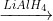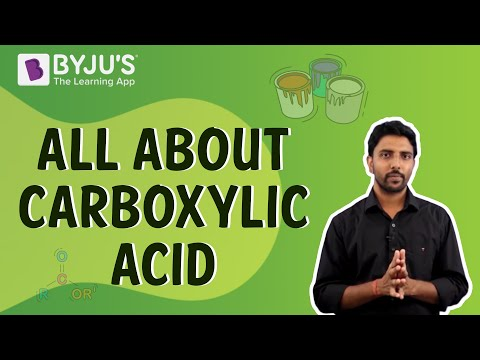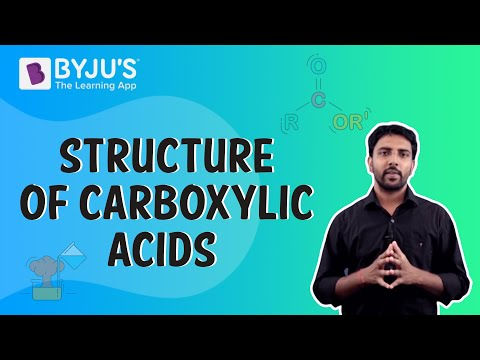Jet Set Go! All about Aeroplanes Jet Set Go! All about Aeroplanes

# Carboxylic Acid Formula Questions

Carboxylic Acids are a class of organic compounds that contain a hydrocarbon parent chain structure linked to a carboxylic acid group. In Carboxylic Acids, one or more carbon atom(s) are attached to an oxygen atom with a double bond and a hydroxyl group with a single bond. The fourth bond of this carbon atom is satisfied either by a hydrogen atom or is attached to the carbon chain.

 Definition: In a Carboxylic Acid Formula, a carboxylic group (COOH) is attached to an alkyl group (R).

## Carboxylic Acid Formula Questions with Solutions

Q1: Write the General Formula of the open-chain Carboxylic Acids.

Answer: The General Formula of open Carboxylic Acids is CnH2n+1COOH.

Where n = number of carbon atoms present in the molecule – 1.

Q2. Which acid is present in the red ants?

Answer: Red ants contain Formic Acid, which is why the bite of a red ant causes itching and irritation.

Q3. Write the name of the following acid: HOOC-COOH.

Answer: The given acid has a molecular formula, C2H2O4. This is Oxalic Acid, also known as Ethanedioic Acid. This acid is commonly found in many plants and vegetables. It is the simplest dicarboxylic acid.

Q4. Explain the reason for the acidity of Carboxylic Acids.

Answer: Carboxylic Acids are acidic because the hydrogen atom in the carboxylic acid group is directly attached to the highly electronegative oxygen atom. Due to this reason, the hydrogen atom can easily be lost in the solution leading to the formation of a hydrogen ion (H+) and a carboxylate anion (RCOO). The number of Hydrogen ions released in the solution leads to the acidity of the solution.

Q5. List the first 5 Carboxylic Acids.

Answer: The first 5 Carboxylic acids contain one, two, three, four, and five carbon atoms in their structure. The general formula of all Carboxylic Acids is CnH2n+1COOH.

Where n = number of carbon atoms present in the molecule -1.

The first five Carboxylic Acids are Methanoic Acid (HCOOH), Ethanoic Acid (CH3COOH), Propanoic Acid (CH3CH2COOH), Butanoic Acid (CH3CH2CH2COOH), and Pentanoic Acid (CH3CH2CH2CH2COOH).

Q6. The Carboxylic Acid that has the highest boiling point is

1. Heptanoic Acid (C6H13COOH)
2. Octanoic Acid (C7H15COOH)
3. Nonanoic Acid (C8H17COOH)
4. Decanoic Acid (C9H19COOH)

Explanation: The intermolecular forces of attraction increase with an increase in the molecular weight of the molecule. From the above-given options, Decanoic Acid has the highest molecular weight and thus has the strongest intermolecular forces. This is why it will require the highest energy to break apart its bonds.

Q7. The compound that is most soluble in Water (H2O) is ______.

1. Pentane
2. Pentanone
3. Ethanoic Acid
4. Pentanoic Acid

Explanation: Water is a polar compound. Thus, it can dissolve only polar molecules in itself. Both Pentane and Pentanone are non-polar compounds. Even though both Ethanoic Acid and Pentanoic Acid are polar in nature, Ethanoic Acid is more soluble in water than Pentanoic Acid. Pentanoic Acid has a larger non-polar carbon chain than Ethanoic Acid. This difference in the size of the non-polar carbon chain makes Ethanoic Acid more soluble in water than Pentanoic Acid.

Q8. List five important uses of Carboxylic Acids.

Answer: Carboxylic Acids are used in various applications, such as in the formation of:

1. Coatings
2. Polymer Formation
4. Drugs
5. Biopolymers

Carboxylic Acids can also be used as solvents, food additives, anti-microbial substances and food flavouring substances.

Q9. Are Carboxylic acids weak or strong?

Answer: Carboxylic Acids are weak acids. This is because they do not ionise completely in water, i.e. the hydrogen ion (H+) concentration in the solution of carboxylic acids is less compared to that in the mineral acids.

Q10. Which Carboxylic Acid is present in human blood?

Answer: Carbonic Acid is present in the human blood and helps in the process of respiration. Even though this acid is present naturally in the human bloodstream, it is advised not to come in contact directly with the concentrated form of this acid, as it may cause irritation to the respiratory tract and the eyes.

Q11. The strongest Carboxylic Acid among the following is:

1. Ethanoic Acid
2. Propanoic Acid
3. Benzoic Acid
4. None of the above

Explanation: Benzoic acid is the strongest. This is due to the delocalisation of electrons within the benzene ring. The delocalisation of electrons within the ring gives different resonating structures. Due to this reason, the benzoate ion is very stable, and the benzoic acid is very strongly acidic.

Q12. The product formed upon the reduction of CH2=CH-COOH with LiAlH4 is:

1. CH3CH2CH2OH
2. CH3CH2COOH
3. CH2=CHCH2OH
4. None of the above

Explanation: Even though LiAlH4 is a strong reducing agent, it cannot reduce the double bond. So, the product formed upon the reduction of CH2=CH-COOH with LiAlH4 is CH2=CHCH2OH.

CH2=CH-COOHCH2=CHCH2OH

Q13. Of the following, the strongest acid is:

1. o-NO2C6H4COOH
2. p-NO2C6H4COOH
3. m-NO2C6H4COOH
4. PhCOOH

Explanation: Due to the ortho-effect, o-NO2C6H4COOH is the strongest of the given acids.

Q14. The acid with the lowest pKa value is:

1. CH3COOH
2. CH3CH2COOH
3. HCOOH
4. (CH3)2COOH

Explanation: pKa for a given acid is the opposite of its acidic strength, i.e. the stronger the acid, the smaller the value of pKa. The smaller the -R group attached to the carboxylic acid group (-COOH), the stronger the acid. Hence, out of the given acids, HCOOH is the strongest acid. Therefore, HCOOH has the lowest pKa value.

Q15. The General Formula CnH2nO2 is for the open-chain:

1. Ketones
2. Aldehydes
3. Alcohols
4. Carboxylic Acids

Explanation: The General formula for carboxylic acids is CnH2n+1COOH.

Where n = number of carbon atoms present in the molecule -1.

On putting n = n-1 in this general formula, the formula becomes:

C(n-1)H2(n-1) + 1COOH = C(n-1)H2n – 2 + 1C1O1O1H1 = CnH2nO2

Thus, the given General Formula is for the open-chain Carboxylic Acids. Thus, option (d) is correct.

## Practice Questions on Carboxylic Acid Formula

Q1. The strongest acid among the following is:

1. HCOOH
2. CH3CH2CH(Cl)COOH
3. CH3COOH
4. CH2(Cl)CH2CH2COOH

Q2. Arrange the following acids in the increasing order of their acid strengths.

(CH3)2CHCOOH, CH3CH(Br)CH2COOH, CH3CH2CH(Br)COOH

Q3. Write a balanced chemical equation for the reaction between CH3COOH and PCl5.

Q4. Write the IUPAC name of the following compound: HOOC – CH = CH – COOH

Q5. Write an equation for the following conversion:

Butanol → Butanoic Acid

Click the PDF to check the answers for Practice Questions.

## Recommended Videos